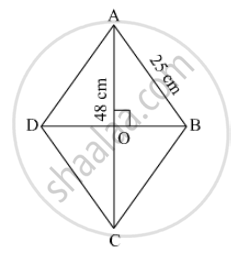SSC (English Medium) Class 8Maharashtra State Board
Share

# If Perimeter of a Rhombus is 100 Cm and Length of One Diagonal is 48 Cm, What is the Area of the Quadrilateral ? - SSC (English Medium) Class 8 - Mathematics

#### Question

If perimeter of a rhombus is 100 cm and length of one diagonal is 48 cm, what is the area of the quadrilateral ?

#### SolutionPerimeter of the rhombus = 100 cm

⇒ 4 xx side = 100

⇒ side = 100/4 = 25cm

Thus, each side of the rhombus = 25 cm.
Diagonals of a rhombus bisect each other at 90°
So, AO = OC = 48/2 = 24 cm

In Δ AOB

We apply Pythagoras theorem,

AO² + OB² = AB²

⇒ 24²  + OB²  = 25²

⇒ OB²  = 625 - 576 = 49

⇒ OB = 7 cm

So, DB = 2 xx "OB" = 2 xx 7 = 14 cm

Area of rhombus = 1/2 xx (product of diagnols) = 1/2 xx 14 xx 48 = 336 cm²

Is there an error in this question or solution?

#### APPEARS IN

Balbharati Solution for Balbharati Class 8 Mathematics (2019 to Current)
Chapter 15: Area
Practice Set 15.2 | Q: 3 | Page no. 97
Solution If Perimeter of a Rhombus is 100 Cm and Length of One Diagonal is 48 Cm, What is the Area of the Quadrilateral ? Concept: Area of a Rhombus.
S